# Lesson 3 - Feature Engineering

These are my personal notes from fast.ai machine learning course and will continue to be updated and improved if I find anything useful and relevant while I continue to review the course to study much more in-depth. Thanks for reading and happy learning!

## Topics​

• Collaborative filtering
• Favorita grocery sales forecasting notebook
• Random forest model interpretation
• Feature importance
• Data leakage
• Collinearity

## Assignments​

• Entering to Grocery Sales Forecasting Kaggle competition

## My Notes​

### What is covered in today's lesson:​

Understanding the data better by using machine learning

• This idea is contrary to the common refrain that things like random forests are black boxes that hide meaning from us. The truth is quite the opposite. Random forests allow us to understand our data deeper and more quickly than traditional approaches.

How to look at larger datasets

Dataset with over 100 million rows—Grocery Forecasting

❓ When to use random forests [00:02:41]?

Cannot think of anything offhand that it is definitely not going to be at least somewhat useful. So it is always worth trying. The real question might be in what situation should we try other things as well, and the short answer to that is for unstructured data (image, sound, etc), you almost certainly want to try deep learning. For collaborative filtering model (groceries competition is of that kind), neither random forest nor deep learning approach is exactly what you want and you need to do some tweaks.

### Review of last week [00:04:42]​

Reading CSV took a minute or two, and we saved it to a feather format file. Feather format is almost the same format that it lives in RAM, so it is ridiculously fast to read and write. The first thing we do is in the lesson 2 notebook is to read in the feather format file.

#### `proc_df` issue [00:05:28]​

An interesting little issue that was brought up during the week is in `proc_df` function. `proc_df` function does the following:

• Finds numeric columns which have missing values and create an additional boolean column as well as replacing the missing with medians.
• Turn the categorical objects into integer codes.

Problem #1: Your test set may have missing values in some columns that were not in your training set or vice versa. If that happens, you are going to get an error when you try to do the random forest since the "missing" boolean column appeared in your training set but not in the test set.

Problem #2: Median of the numeric value in the test set may be different from the training set. So it may process it into something which has different semantics.

Solution: There is now an additional return variable `nas` from `proc_df` which is a dictionary whose keys are the names of the columns that had missing values, and the values of the dictionary are the medians. Optionally, you can pass `nas` to `proc_df` as an argument to make sure that it adds those specific columns and uses those specific medians:

``df, y, nas = proc_df(df_raw, 'SalePrice', nas)``

### Corporación Favorita Grocery Sales Forecasting [00:09:25]​

Let's walk through the same process when you are working with a really large dataset. It is almost the same but there are a few cases where we cannot use the defaults because defaults run a little bit too slowly.

It is important to be able to explain the problem you are working on. The key things to understand in a machine learning problem are:

• What are the independent variables?
• What is the dependent variable (the thing you are trying to predict)?

In this competition

• Dependent variable—how many units of each kind of product were sold in each store on each day during the two week period.
• Independent variables—how many units of each product at each store on each day were sold in the last few years. For each store, where it is located and what class of store it is (metadata). For each type of product, what category of product it is, etc. For each date, we have metadata such as what the oil price was.

This is what we call a relational dataset. Relational dataset is one where we have a number of different pieces of information that we can join together. Specifically this kind of relational dataset is what we refer to as "star schema" where there is some central transactions table. In this competition, the central transactions table is `train.csv` which contains the number units that were sold by `date` , `store_nbr` , and `item_nbr`. From this, we can join various bits of metadata (hence the name "star" schema — there is also one called "snowflake" schema).

``types = {'id': 'int64',         'item_nbr': 'int32',         'store_nbr': 'int8',         'unit_sales': 'float32',         'onpromotion': 'object'}%%timedf_all = pd.read_csv(f'{PATH}train.csv', parse_dates=['date'],                     dtype=types, infer_datetime_format=True)# -----------------------------------------------------------------------------# Output# -----------------------------------------------------------------------------CPU times: user 1min 41s, sys: 5.08s, total: 1min 46sWall time: 1min 48s``
• If you set `low_memory=False`, it will run out of memory regardless of how much memory you have.
• In order to limit the amount of space that it takes up when you read in, we create a dictionary for each column name to the data type of that column. It is up to you to figure out the data types by running or `less` or `head` on the dataset.
• With these tweaks, we can read in 125,497,040 rows in less than 2 minutes.
• Python itself is not fast, but almost everything we want to do in Python in data science has been written for us in C or more often in Cython which is a python like language that compiles to C. In Pandas, a lot of it is written in assembly language which is heavily optimized. Behind the scene, a lot of that is going back to calling Fortran based libraries for linear algebra.

❓ Are there any performance consideration to specifying `int64` vs. `int` [00:18:33]?

The key performance here was to use the smallest number of bits that I could to fully represent the column. If we used `int8` for `item_nbr` , the maximum `item_nbr` is bigger than 255 and it will not fit. On the other hand, if we used `int64` for the `store_nbr` , it is using more bits than necessary. Given that the whole purpose here was to avoid running out of RAM, we do not want to use up 8 times more memory than necessary. When you are working with large datasets, very often you will find that the slow piece is reading and writing to RAM, not the CPU operations. Also as a rule of thumb, smaller data types often will run faster particularly if you can use Single Instruction Multiple Data (SIMD) vectorized code, it can pack more numbers into a single vector to run at once.

❓ Do we not have to shuffle the data anymore [00:20:11]?

Although here I have read in the whole thing, when I start I never start by reading in the whole thing. By using a UNIX command `shuf`, you can get a random sample of data at the command prompt and then you can just read that. This is a good way, for example, to find out what data types to use — read in a random sample and let Pandas figure it out for you. In general, I do as much work as possible on a sample until I feel confident that I understand the sample before I move on.

To pick a random line from a file using `shuf` use the `-n` option. This limits the output to the number specified. You can also specify the output file:

`shuf -n 5 -o sample_training.csv train.csv`

`'onpromotion': ‘object'` [00:21:28]—`object` is a general purpose Python datatype which is slow and memory heavy. The reason for this is it is a boolean which also has missing values, so we need to deal with this before we can turn it into a boolean as you see below:

``df_all.onpromotion.fillna(False, inplace=True)df_all.onpromotion = df_all.onpromotion.map({'False': False,                                             'True': True})df_all.onpromotion = df_all.onpromotion.astype(bool)%time df_all.to_feather('tmp/raw_groceries')``
• `fillna(False)`: we would not do this without checking first, but some exploratory data analysis shows that it is probably an appropriate thing to do (i.e. missing means false).
• `map({‘False': False, ‘True': True})` : `object` usually reads in as string, so replace string `‘True'` and `‘False'` with actual booleans.
• `astype(bool)` : Then finally convert it to boolean type.
• The feather file with over 125 million records takes up something under 2.5GB of memory.
• Now it is in a nice fast format, we can save it to feather format in under 5 seconds.

Pandas is generally fast, so you can summarize every column of all 125 million records in 20 seconds:

``%time df_all.describe(include='all')``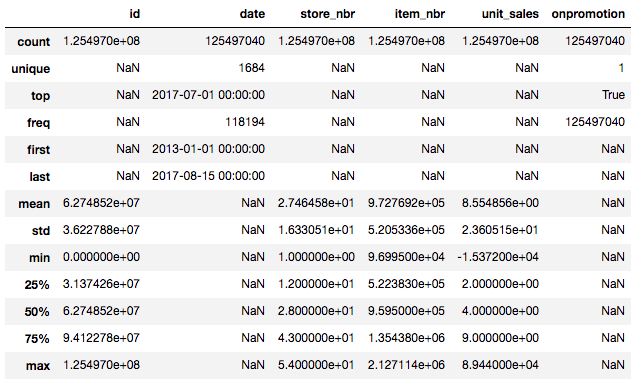• First thing to look at is the dates. Dates are important because any models you put in in practice, you are going to be putting it in at some date that is later than the date that you trained it by definition. So if anything in the world changes, you need to know how your predictive accuracy changes as well. So for Kaggle or for your own project, you should always make sure that your dates do not overlap [00:22:55].
• In this case, training set goes from 2013 to August 2017.
``df_test = pd.read_csv(f'{PATH}test.csv', parse_dates = ['date'],                      dtype=types, infer_datetime_format=True)df_test.onpromotion.fillna(False, inplace=True)df_test.onpromotion = df_test.onpromotion.map({'False': False,                                               'True': True})df_test.onpromotion = df_test.onpromotion.astype(bool)df_test.describe(include='all')``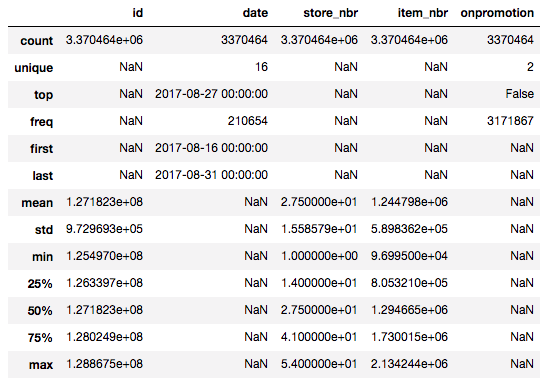• In our test set, they go from one day later until the end of the month.
• This is a key thing — you cannot really do any useful machine learning until you understand this basic piece. You have four years of data and you are trying to predict the next two weeks. This is a fundamental thing you need to understand before you can go and do a good job at this.
• If you want to use a smaller dataset, we should use the most recent — not random set.

❓ Wouldn't four years ago around the same time frame be important (e.g. around Christmas time)[00:25:06]?

Exactly. It is not that there is no useful information from four years ago so we do not want to entirely throw it away. But as a first step, if you were to submit the mean, you would not submit the mean of 2012 sales, but probably want to submit the mean of last month's sale.And later on, we might want to weight more recent dates more highly since they are probably more relevant. But we should do bunch of exploratory data analysis to check that.

``df_all.tail()``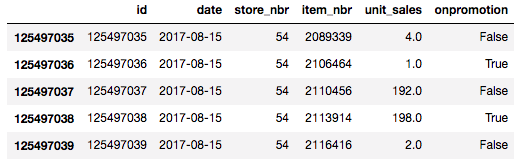Here is what the bottom of the data looks like [00:26:00].

``df_all.unit_sales = np.log1p(np.clip(df_all.unit_sales, 0, None))``
• We have to take a log of the sales because we are trying to predict something that varies according to the ratios and they told us, in this competition, that root mean squared log error is something they care about.
• `np.clip(df_all.unit_sales, 0, None)` : there are some negative sales that represent returns and the organizer told us to consider them to be zero for the purpose of this competition. `clip` truncates to specified min and max.
• `np.log1p` : log of the value plus 1. The competition detail tells you that they are going to use root mean squared log plus 1 error because log(0) does not make sense.
``%time add_datepart(df_all, 'date')# -----------------------------------------------------------------------------# Output# -----------------------------------------------------------------------------CPU times: user 1min 35s, sys: 16.1 s, total: 1min 51sWall time: 1min 53s``

We can add date part as usual. It takes a couple of minutes, so we should run through all this on sample first to make sure it works. Once you know everything is reasonable, then go back and run on a whole set.

``n_valid = len(df_test)n_trn = len(df_all) - n_validtrain, valid = split_vals(df_all, n_trn)train.shape, valid.shape# -----------------------------------------------------------------------------# Output# -----------------------------------------------------------------------------((122126576, 18), (3370464, 18))``

These lines of code are identical to what we saw for bulldozers competition. We do not need to run `train_cats` or `apply_cats` since all of the data types are already numeric (remember `apply_cats` applies the same categorical codes to validation set as the training set) [00:27:59].

``%%timetrn, y, nas  = proc_df(train, 'unit_sales')val, y_val, nas = proc_df(valid, 'unit_sales', nas)``

Call proc_df to check the missing values and so forth.

``def rmse(x,y): return math.sqrt(((x-y)**2).mean())def print_score(m):    res = [rmse(m.predict(X_train), y_train),           rmse(m.predict(X_valid), y_valid),           m.score(X_train, y_train), m.score(X_valid, y_valid)]    if hasattr(m, 'oob_score_'): res.append(m.oob_score_)    print(res)``

These lines of code again are identical. Then there are two changes [00:28:48]:

``set_rf_samples(1_000_000)%time x = np.array(trn, dtype=np.float32)CPU times: user 1min 17s, sys: 18.9 s, total: 1min 36sWall time: 1min 37sm = RandomForestRegressor(n_estimators=20, min_samples_leaf=100,                          n_jobs=8)%time m.fit(x, y)``

We have learned about `set_rf_samples` last week. We probably do not want to create a tree from 125 million records (not sure how long that will take). You can start with 10k or 100k and figure out how much you can run. There is no relationship between the size of the dataset and how long it takes to build the random forests — the relationship is between the number of estimators multiplied by the sample size.

❓ What is `n_job`?

In the past, it has always been `-1` [00:29:42]. The number of jobs is the number of cores to use. I was running this on a computer that has about 60 cores and if you try to use all of them, it spent so much time spinning out jobs and it was slower. If you have lots of cores on your computer, sometimes you want less (`-1` means use every single core).

Another change was `x = np.array(trn, dtype=np.float32)`. This converts data frame into an array of floats and we fit it on that. Inside the random forest code, they do this anyway. Given that we want to run a few different random forests with a few different hyper parameters, doing this once myself saves 1 min 37 sec.

### Profiler : `%prun` [00:30:40]​

If you run a line of code that takes quite a long time, you can put `%prun` in front.

``%prun m.fit(x, y)``
• This will run a profiler and tells you which lines of code took the most time. Here it was the code in scikit-learn that was the line of code that converts data frame to numpy array.
• Looking to see which things is taking up the time is called "profiling" and in software engineering is one of the most important tool. But data scientists tend to under appreciate it.
• For fun, try running `%prun` from time to time on code that takes 10–20 seconds and see if you can learn to interpret and use profiler outputs.
• Something else Jeremy noticed in the profiler is we can't use OOB score when we do `set_rf_samples` because if we do, it will use the other 124 million rows to calculate the OOB score. Besides, we want to use the validation set that is the most recent dates rather than random.
``print_score(m)# -----------------------------------------------------------------------------# Output# -----------------------------------------------------------------------------[0.7726754289860, 0.7658818632043, 0.23234198105350, 0.2193243264]``

So this got us 0.76 validation root mean squared log error.

``m = RandomForestRegressor(n_estimators=20, min_samples_leaf=10, n_jobs=8)%time m.fit(x, y)``

This gets us down to 0.71 even though it took a little longer.

``m = RandomForestRegressor(n_estimators=20, min_samples_leaf=3, n_jobs=8)%time m.fit(x, y)``

This brought this error down to 0.70. `min_samples_leaf=1` did not really help. So we have a "reasonable" random forest here. But this does not give a good result on the leader board [00:33:42]. Why? Let's go back and see the data: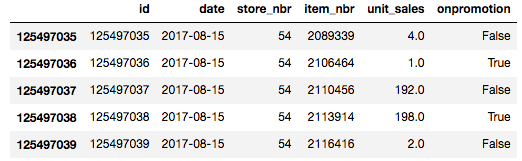These are the columns we had to predict with (plus what were added by `add_datepart`). Most of the insight around how much of something you expect to sell tomorrow is likely to be wrapped up in the details about where the store is, what kind of things they tend to sell at the store, for a given item, what category of item it is. Random forest has no ability to do anything other than create binary splits on things like day of week, store number, item number. It does not know type of items or location of stores. Since its ability to understand what is going on is limited, we probably need to use the entire 4 years of data to even get some useful insights. But as soon as we start using the whole 4 years of data, a lot of the data we are using is really old. There is a Kaggle kernel that points out that what you could do is [00:35:54]:

1. Take the last two weeks.
2. Take the average sales by store number, by item number, by on promotion, then take a mean across date.
3. Just submit that, and you come about 30th 🎉

We will talk about this in the next class, but if you can figure out how you start with that model and make it a little bit better, you will be above 30th place.

❓ Could you try to capture seasonality and trend effects by creating new columns like average sales in the month of August [00:38:10]?

It is a great idea. The thing to figure out is how to do it because there are details to get right and are difficult- not intellectually difficult but they are difficult in a way that makes you headbutt your desk at 2am [00:38:41].

Coding you do for machine learning is incredibly frustrating and incredibly difficult. If you get a detail wrong, much of the time it is not going to give you an exception it will just silently be slightly less good than it otherwise would have been. If you are on Kaggle, you will know that you are not doing as well as other people. But otherwise you have nothing to compare against. You will not know if your company's model is half as good as it could be because you made a little mistake. This is why practicing on Kaggle now is great.

You will get practice in finding all of the ways in which you can infuriatingly screw things up and you will be amazed [00:39:38].

Even for Jeremy, there is an extraordinary array of them. As you get to get to know what they are, you will start to know how to check for them as you go. You should assume every button you press, you are going to press the wrong button. That is fine as long as you have a way to find out.

Unfortunately there is not a set of specific things you should always do, you just have to think what you know about the results of this thing I am about to do. Here is a really simple example. If you created that basic entry where you take the mean by date, by store number, by on promotion, you submitted it, and got a reasonable score. Then you think you have something that is a little bit better and you do predictions for that. How about you create a scatter plot showing the prediction of your average model on one axis versus the predictions of your new model on the other axis. You should see that they just about form a line. If they do not, then that is a very strong suggestion that you screwed something up.

❓ How often do you pull in data from other sources to supplement dataset you have [00:41:15]?

Very often. The whole point of star schema is that you have a centric table, and you have other tables coming off it that provide metadata about it. On Kaggle, most competitions have the rule that you can use external data as long as post on the forum and is publicly available (double check the rule!). Outside of the Kaggle, you should always be looking for what external data you could possibly leverage.

That information is actually provided. In general, one way of tackling this kind of problem is to create lots of new columns containing things like average number of sales on holidays, average percent change in sale between January and February, etc. There has been a previous competition for a grocery chain in Germany that was almost identical. The person who won was a domain expert and specialist in doing logistics predictions. He created lots of columns based on his experience of what kinds of things tend to be useful for making predictions. So that is an approach that can work. The third place winner did almost no feature engineering, however, and they also had one big oversight which may have cost them the first place win. We will be learning a lot more about how to win this competition and ones like it as we go.

### Importance of good validation set [00:44:53]​

If you do not have a good validation set, it is hard, if not impossible, to create a good model. If you are trying to predict next month's sales and you build models. If you have no way of knowing whether the models you have built are good at predicting sales a month ahead of time, then you have no way of knowing whether it is actually going to be any good when you put your model in production. You need a validation set that you know is reliable at telling you whether or not your model is likely to work well when you put it in production or use it on the test set.

Normally you should not use your test set for anything other than using it right at the end of the competition or right at the end of the project to find out how you did. But there is one thing you can use the test set for in addition — that is to calibrate your validation set [00:46:02].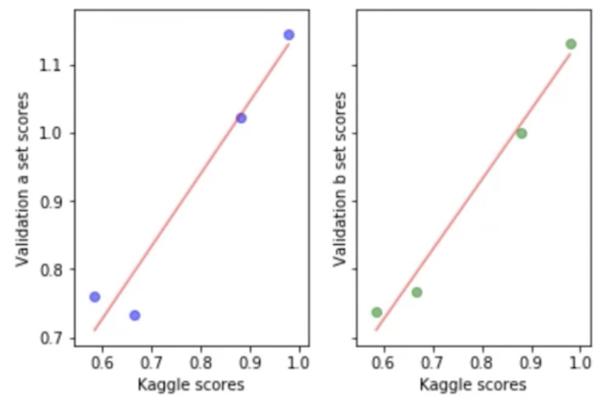What Terrance did here was that he built four different models and submitted each of the four models to Kaggle to find out its score. X-axis is the score Kaggle told us on the leaderboard, and y-axis he plotted the score on a particular validation set he was trying out to see whether the validation set was going to be any good. If your validation set is good, then the relationship between the leaderboards score (i.e. the test set score) should lie in a straight line. Ideally, it will lie on the `y = x` line, but honestly that does not matter too much as long as relatively speaking it tells you which models are better than which other models, then you know which model is the best. In this case, Terrance has managed to come up with a validation set which looks like it is going to predict the Kaggle leaderboard score well. That is really cool because he can go away and try a hundred different types of models, feature engineering, weighting, tweaks, hyper parameters, whatever else, see how they go on the validation set, and not have to submit to Kaggle. So you will get a lot more iterations, a lot more feedback. This is not just true for Kaggle but every machine learning project you do. In general, if your validation set is not showing nice fit line, you need think carefully [00:48:02]. How is the test set constructed? How is my validation set different? You will have to draw lots of charts and so forth to find out.

❓ How do you construct a validation set as close to the test set [00:48:23]?

Here are a few tips from Terrance:

• Close by date (i.e. most recent)
• First looked at the date range of the test set (16 days), then looked at the date range of the kernel which described how to get 0.58 on the leaderboard by taking an average (14 days).
• Test set begins on the day after pay day and ends on a pay day.
• Plot lots of pictures. Even if you did not know it was pay day, you want to draw the time series chart and hopefully see that every two weeks there is a spike and make sure that you have the same number of spikes in the validation set as the test set.

### Interpreting machine learning models [00:50:38]​

Notebook

``PATH = "data/bulldozers/"df_raw = pd.read_feather('tmp/raw')df_trn, y_trn, nas = proc_df(df_raw, 'SalePrice')``

We start by reading in our feather files for Blue Books for Bulldozers competition. Reminder: we have already read in the CSV, processed it into categories, and save it in feather format. The next thing we do is call `proc_df` to turn categories into integers, deal with missing values, and pull out the dependent variable. Then create a validation set just like last week:

``def split_vals(a, n): return a[:n], a[n:]n_valid = 12000n_trn = len(df_trn) - n_validX_train, X_valid = split_vals(df_trn, n_trn)y_train, y_valid = split_vals(y_trn, n_trn)raw_train, raw_valid = split_vals(df_raw, n_trn)``

#### Detour to lesson 1 notebook [00:51:59]​

Last week, there was a bug in `proc_df` that was shuffling the dataframe when `subset` gets passed in hence causing the validation set to be not the latest 12000 records. This issue was fixed.

``## From lesson1-rf.ipynbdf_trn, y_trn, nas = proc_df(df_raw, 'SalePrice', subset=30000, na_dict=nas)X_train, _ = split_vals(df_trn, 20000)y_train, _ = split_vals(y_trn, 20000)``

❓ Why is `nas` both input and output of this function [00:53:03]?

`proc_df` returns a dictionary telling you which columns were missing and for each of those columns what the median was.

1. When you call `proc_df` on a larger dataset, you do not pass in `nas` but you want to keep that return value.
2. Later on, when you want to create a subset (by passing in `subset`), you want to use the same missing columns and medians, so you pass nas in.
3. If it turns out that the subset was from a whole different dataset and had different missing columns, it would update the dictionary with additional key value.
4. It keeps track of any missing columns you came across in anything you passed to `proc_df` .

#### Back to lesson 2 notebook [00:54:40]​

Once we have done `proc_df`, this is what it looks like. `SalePrice` is the log of the sale price.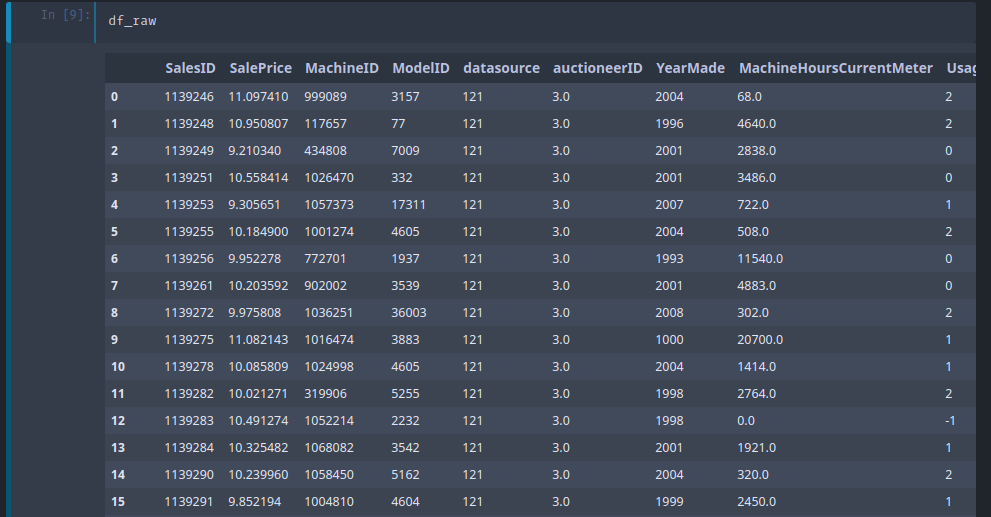We already know how to get the prediction. We take the average value in each leaf node in each tree after running a particular row through each tree. Normally, we do not just want a prediction — we also want to know how confident we are of that prediction.

We would be less confident of a prediction if we have not seen many examples of rows like this one. In that case, we would not expect any of the trees to have a path through — which is designed to help us predict that row. So conceptually, you would expect then that as you pass this unusual row through different trees, it is going to end up in very different places. In other words, rather than just taking the mean of the predictions of the trees and saying that is our prediction, what if we took the standard deviation of the predictions of the trees? If the standard deviation is high, that means each tree is giving us a very different estimate of this row's prediction. If this was a really common kind of row, the trees would have learned to make good predictions for it because it has seen lots of opportunities to split based on those kind of rows. So the standard deviation of the predictions across the trees gives us at least relative understanding of how confident we are of this prediction [00:56:39]. This is not something which exists in scikit-learn, so we have to create it. But we already have almost the exact code we need.

For model interpretation, there is no need to use the full dataset because we do not need a massively accurate random forest — we just need one which indicates the nature of relationships involved.

Just make sure the sample size is large enough that if you call the same interpretation commands multiple times, you do not get different results back each time. In practice, 50,000 is a high number and it would be surprising if that was not enough (and it runs in seconds).

``set_rf_samples(50000)m = RandomForestRegressor(n_estimators=40, min_samples_leaf=3,                        max_features=0.5, n_jobs=-1, oob_score=True)m.fit(X_train, y_train)print_score(m)# -----------------------------------------------------------------------------# Output# -----------------------------------------------------------------------------[0.20538495115059174, 0.2463770070315658, 0.911839902018488, 0.8915952469905479, 0.8948441803136404]``

Here is where we can do the exact same list comprehension as the last time [00:58:35]:

``%time preds = np.stack([t.predict(X_valid) for t in m.estimators_])np.mean(preds[:, 0]), np.std(preds[:, 0])# -----------------------------------------------------------------------------# Output# -----------------------------------------------------------------------------CPU times: user 1.39 s, sys: 32 ms, total: 1.42 sWall time: 1.43 s(9.363183303035791, 0.29177819981067427)``

This is how to do it for one observation. This takes quite a while and specifically, it is not taking advantage of the fact that my computer has lots of cores in it. List comprehensions itself is Python code and Python code (unless you are doing something special) runs in serial which means it runs on a single CPU and does not take advantage of your multi CPU hardware. If we wanted to run this on more trees and more data, the execution time goes up. Wall time (the amount of actual time it took) is roughly equal to the CPU time where else if it was running on lots of cores, the CPU time would be higher than the wall time [01:00:05].

It turns out fastai library provides a handy function called `parallel_trees`:

``def get_preds(t): return t.predict(X_valid)%time preds = np.stack(parallel_trees(m, get_preds))np.mean(preds[:, 0]), np.std(preds[:, 0])# -----------------------------------------------------------------------------# Output# -----------------------------------------------------------------------------CPU times: user 100 ms, sys: 180 ms, total: 280 msWall time: 505 ms(9.1960278072006023, 0.21225113407342761)``
• `parallel_trees` takes a random forest model `m` and some function to call (here, it is `get_preds`). This calls this function on every tree in parallel.
• It will return a list of the result of applying that function to every tree.
• This will cut down the wall time to 500 milliseconds and giving exactly the same answer. Time permitting, we will talk about more general ways of writing code that runs in parallel which is super useful for data science, but here is one that we can use for random forests.

#### Plotting [01:02:02]​

We will first create a copy of the data and add the standard deviation of the predictions and predictions themselves (the mean) as new columns:

``x = raw_valid.copy()x['pred_std'] = np.std(preds, axis=0)x['pred'] = np.mean(preds, axis=0)x.Enclosure.value_counts().plot.barh()``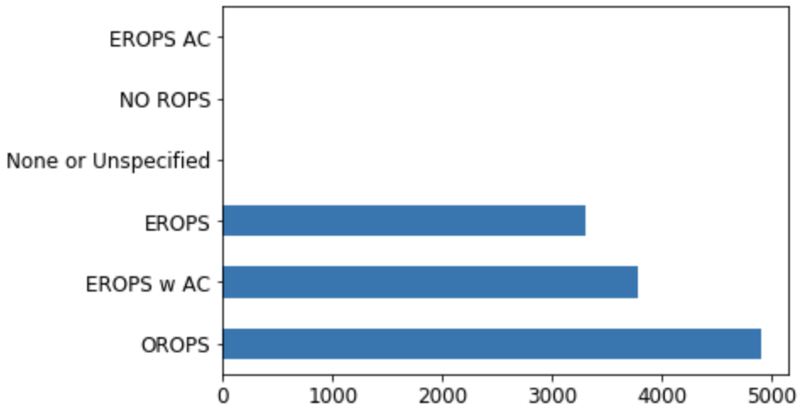You might remember from last lesson that one of the predictors we have is called `Enclosure` and this is an important one as we will see later. Let's start by doing a histogram. One of the nice things about Pandas is it has built-in plotting capabilities.

❓ Can you remind me what enclosure is [01:02:50]?

We do not know what it means and it does not matter. The whole purpose of this process is that we are going to learn about what things are (or at least what things are important and later on figure out what they are and how they are important). So we will start out knowing nothing about this dataset. We are just going to look at something called `Enclosure` that has something called `EROPS` and `ROPS` and we do not even know what this is yet. All we know is that the only three that appear in any great quantity are `OROPS`, `EROPS w AC`, and `EROPS`. This is very common as a data scientist. You often find yourself looking at data that you are not that familiar with and you have to figure out which bits to study more carefully, which bits seem to matter, and so forth. In this case, at least know that `EROPS AC`, `NO ROPS`, and `None or Unspecified` we really do not care about because they basically do not exist. So we will focus on `OROPS`, `EROPS w AC`, and `EROPS`.

Here we took our data frame, grouped by `Enclosure`, then took average of 3 fields [01:04:00]:

``flds = ['Enclosure', 'SalePrice', 'pred', 'pred_std']enc_summ = x[flds].groupby('Enclosure', as_index=False).mean()enc_summ``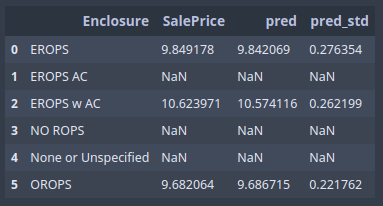We can already start to learn a little here:

• Prediction and the sale price are close to each other on average (good sign)
• Standard deviation varies a little bit
``enc_summ = enc_summ[~pd.isnull(enc_summ.SalePrice)]enc_summ.plot('Enclosure', 'SalePrice', 'barh', xlim=(0, 11))``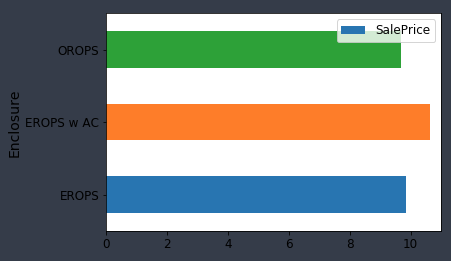``enc_summ.plot('Enclosure', 'pred', 'barh', xerr='pred_std', alpha=0.6, xlim=(0, 11))``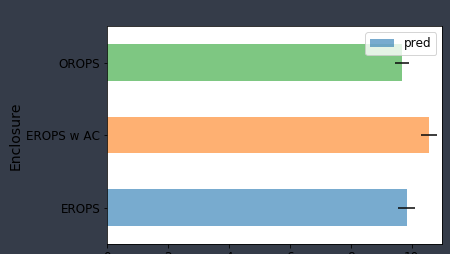We used the standard deviation of prediction for the error bars above. This will tell us if there is some groups or some rows that we are not very confident of at all. We could do something similar for product size:

``raw_valid.ProductSize.value_counts().plot.barh()``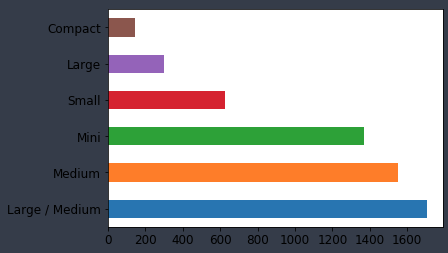``flds = ['ProductSize', 'SalePrice', 'pred', 'pred_std']summ = x[flds].groupby(flds).mean()summ``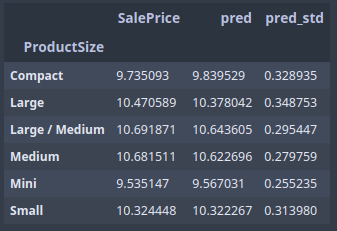You expect, on average, when you are predicting something that is a bigger number your standard deviation would be higher. So you can sort by the ratio of the standard deviation of the predictions to the predictions themselves [01:05:51].

``(summ.pred_std / summ.pred).sort_values(ascending=False)``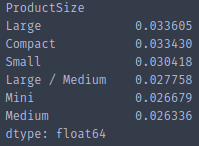What this tells us is that product size `Large` and `Compact` , our predictions are less accurate (relatively speaking as a ratio of the total price). So if we go back and have a look, you see why. These are the smallest groups in the histogram. As you would expect, in small groups, we are doing a less good job.

You can use this confidence interval for two main purposes:

1. You can look at the average confidence interval by group to find out if there are groups you do not seem to have confidence about.
2. Perhaps more importantly, you can look at them for specific rows. When you put it in production, you might always want to see the confidence interval. For example, if you are doing credit scoring to decide whether to give somebody a loan, you probably want to see not only what their level of risk is but how confident we are. If they want to borrow lots of money and we are not at all confident about our ability to predict whether they will pay back, we might want to give them a smaller loan.

### Feature importance [01:07:20]​

I always look at feature importance first in practice. Whether I'm working on a Kaggle competition or a real world project, I build a random forest as fast as I can, trying to get it to the point that is significantly better than random but doesn't have to be much better than that. And the next thing I do is to plot the feature importance.

The feature importance tells us in this random forest, which columns mattered. We have dozens of columns in this dataset, and here, we are picking out the top 10. `rf_feat_importance` is part of fast.ai library which takes a model `m` and dataframe `df_trn` (because we need to know names of columns) and it will give you back a Pandas dataframe showing you in order of importance how important each column was.

``fi = rf_feat_importance(m, df_trn)fi[:10]``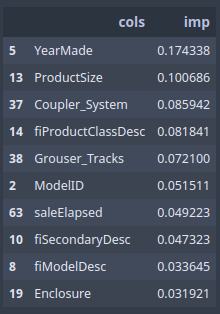``fi.plot('cols', 'imp', figsize=(10, 6), legend=False)``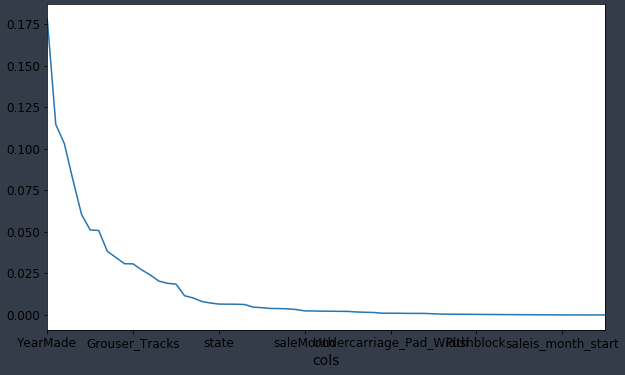Since `fi` is a `DataFrame`, we can use `DataFrame` plotting commands [01:09:00]. The important thing is to see that some columns are really important and most columns do not really matter at all. In nearly every dataset you use in real life, this is what your feature importance is going to look like. There is only a handful of columns that you care about, and this is why Jeremy always starts here. At this point, in terms of looking into learning about this domain of heavy industrial equipment auctions, we only have to care about learning about the columns which matter. Are we going to bother learning about `Enclosure`? Depends whether `Enclosure` is important. It turns out that it appears in top 10, so we are going to have to learn about `Enclosure`.

We can also plot this as a bar plot:

``def plot_fi(fi): return fi.plot('cols', 'imp', 'barh', figsize=(12, 7), legend=False)plot_fi(fi[:30])``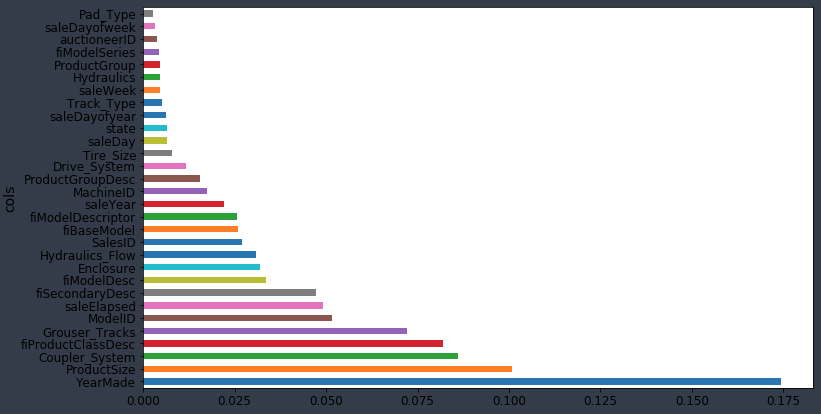The most important thing to do with this is to now sit down with your client, your data dictionary, or whatever your source of information is and say to then "okay, tell me about `YearMade`. What does that mean? Where does it come from?" [01:10:31] Plot lots of things like histogram of `YearMade` and scatter plot of `YearMade` against price and learn everything you can because `YearMade` and `Coupler_System `—they are the things that matter.

What will often happen in real-world projects is that you sit with the the client and you'll say "it turns out the `Coupler_System` is the second most important thing" and they might say "that makes no sense." That doesn't mean that there is a problem with your model, it means there is a problem with their understanding of the data they gave you.

Let me give you an example [01:11:16]. I entered a Kaggle competition where the goal was to predict which applications for grants at a university would be successful. I used this exact approach and I discovered a number of columns which were almost entirely predictive of the dependent variable. Specifically, when I then looked to see in what way they are predictive, it turned out whether they were missing or not was the only thing that mattered in his dataset. I ended up winning that competition thanks to this insight. Later on, I heard what had happened. It turns out that at that university, there is an administrative burden to fill any other database and so for a lot of the grant applications, they do not fill in the database for the folks whose applications were not accepted. In other words, these missing values in the dataset were saying this grand wasn't accepted because if it was accepted then the admin folks will go in and type in that information. This is what we call data leakage. Data leakage means there is information in the dataset that I was modeling with which the university would not have had in real life at that point in time they were making a decision. When they are actually deciding which grant applications to prioritize, they do not know which ones the admin staff will later on going to add information to because it turns out that they were accepted.

One of the key things you will find here is data leakage problems and that is a serious problem you need to deal with [01:12:56]. The other thing that will happen is you will often find its signs of collinearity. It seems like what happened with `Coupler_System`. `Coupler_System` tells you whether or not a particular kind of heavy industrial equipment has a particular feature on it. But if it is not that kind of industrial equipment at all, it will be missing. So it indicates whether or not it is a certain class of heavy industrial equipment. This is not data leakage. This is an actual information you actually have at the right time. You just have to be careful interpreting it. So you should go through at least the top 10 or look for where the natural break points are and really study these things carefully.

To make life easier, it is sometimes good to throw some data away and see if it make any difference. In this case, we have a random forest which was .889 r². Here we filter out those where the importance is equal to or less than 0.005 (i.e. only keep the one whose importance is greater than 0.005).

``to_keep = fi[fi.imp > 0.005].colslen(to_keep)df_keep = df_trn[to_keep].copy()X_train, X_valid = split_vals(df_keep, n_trn)m = RandomForestRegressor(n_estimators=40, min_samples_leaf=3, max_features=0.5,                          n_jobs=-1, oob_score=True)m.fit(X_train, y_train)print_score(m)# -----------------------------------------------------------------------------# Output# -----------------------------------------------------------------------------[0.20685390156773095, 0.24454842802383558, 0.91015213846294174, 0.89319840835270514, 0.8942078920004991]``

The r² did not change much — it actually increased a tiny bit. Generally speaking, removing redundant columns should not make it worse. If it makes it worse, they were not redundant after all. It might make it a little bit better because if you think about how we built these trees, when it is deciding what to split on, it has less things to worry about trying, it is less often going to accidentally find a crappy column. So there is slightly better opportunity to create a slightly better tree with slightly less data, but it is not going to change it by much. But it is going to make it a bit faster and it is going to let us focus on what matters. Let's re-run feature importance on this new result [01:15:49].

``fi = rf_feat_importance(m, df_keep)plot_fi(fi)``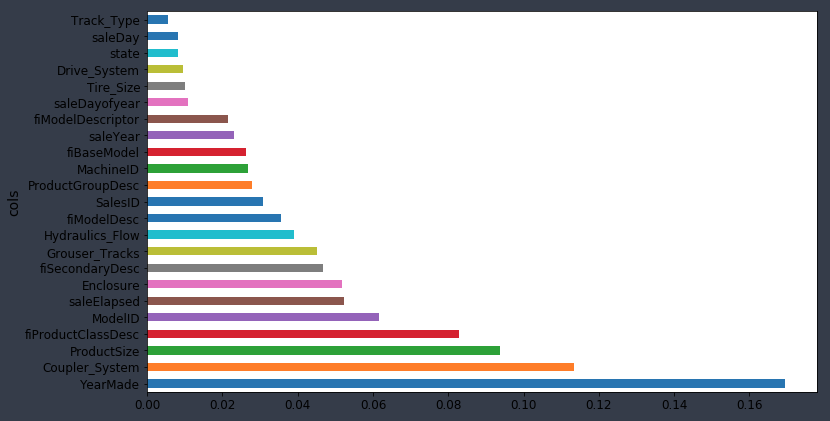Key thing that has happened is that when you remove redundant columns, you are also removing sources of collinearity. In other words, two columns that might be related to each other. Collinearity does not make your random forests less predictive, but if you have a column A is a little bit related to a column B, and B is a strong driver of the independent, what happens is that the importance is going to be split between A and B. By removing some of those columns with very little impact, it makes your feature importance plot clearer. Before `YearMade` was pretty close to `Coupler_System`. But there must have been a bunch of things that are collinear with `YearMade` and now you can see `YearMade` really matters. This feature importance plot is more reliable than the one before because it has a lot less collinearity to confuse us.

#### Let's talk about how this works [01:17:21]​

Not only is it really simple, it is a technique you can use not just for random forests but for basically any kind of machine learning model. Interestingly, almost no one knows this. Many people will tell you there is no way of interpreting this particular kind of model (the most important interpretation of a model is knowing which things are important) and that is almost certainly not going to be true because the technique I am going to teach you actually works for any kind of models.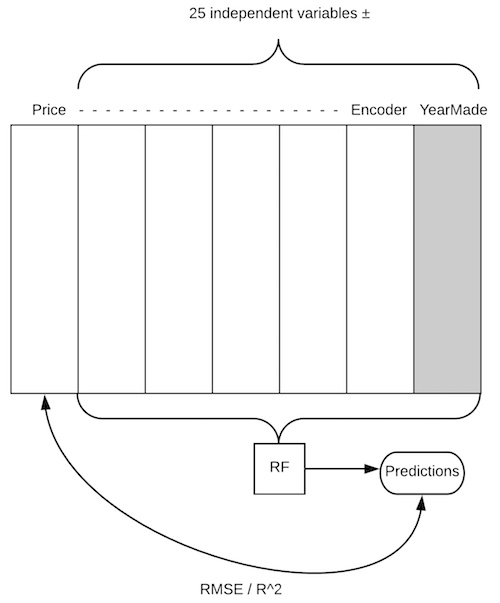• We take our bulldozer data set and we have a column `Price` we are trying to predict (dependent variable).
• We have 25 independent variables and one of them is `YearMade`.
• How do we figure out how important `YearMade` is? We have a whole random forest and we can find out our predictive accuracy. So we will put all these rows through our random forest, and it will spit out some predictions. We will then compare them to the actual price (in this case, we get our root mean squared error and r²). This is our starting point.
• Let's do exactly the same thing, but this time, take the `YearMade` column and randomly shuffle it (i.e. randomly permute just that column). Now `YearMade` has exactly the same distribution as before (same mean, same standard deviation). But it has no relationships with our dependent variable at all because we totally randomly reordered it.
• Before, we might have found our r² was .89. After we shuffle `YearMade`, we check again, and now r² is .80. The score got much worse when we destroyed that variable.
• Okay, let's try again. We put `YearMade` back to how it was, and this time let's take `Enclosure` and shuffle that. This time, r²is .84 and we can say the amount of decrease in our score for `YearMade` was .09 and the amount of decrease for `Enclosure` was .05. And this is going to give us our feature importances for each column.

❓ Can't we just exclude the column and check the decay in performance [01:20:31]?

You could remove the column and train a whole new random forest, but that is going to be really slow. Where else this way, we can keep our random forest and just test the predictive accuracy of it again. So this is nice and fast by comparison. In this case, we just have to rerun every row forward through the forest for each shuffled column.

❓ If you want to do multi-collinearity, would you do two of them and random shuffle and then three of them [01:21:12]?

I don't think you mean multi-collinearity, I think you mean looking for interaction effects. So if you want to say which pairs of variables are most important, you could do exactly the same thing each pair in turn. In practice, there are better ways to do that because that is obviously computationally pretty expensive and so we will try to find time to do that if we can.

We now have a model which is a little bit more accurate and we have learned a lot more about it. So we are out of time and what I would suggest you try doing now before next class for this bulldozers dataset is going through the top 5 or 10 predictors and try and learn what you can about how to draw plots in Pandas and try to come back with some insights about things like:

• what is the relationship between `YearMade` and the dependent variable
• what is the histogram of `YearMade`
• now that you know `YearMade` is really important, check if there is some noise in that column which we could fix
• Check if there is some weird encoding in that column we can fix
• This idea Jeremy had that maybe `Coupler_System` is there entirely because it is collinear with something else, you might want try and figure out if it's true. If so, how would you do it?
• `fiProductClassDesc` that rings alarm bells — it sounds like it might be a high cardinality categorical variable. It might be something with lots and lots levels because it sounds like it is a model name. So go and have a look at that model name — does it have some order into it? Could you make it an ordinal variable to make it better? Does it have some kind of hierarchical structure in the string that we can split it on hyphen to create more sub columns.

Have a think about this. Try and make it so that by when you come back, you've got some new, ideally a better accuracy than what I just showed because you found some new insights or at least that you can tell the class about some things you have learnt about how heavy industrial equipment auctions work in practice.